# 12-267/Homework Assignment 5

This assignment is due in class on Friday November 2. Here and everywhere, neatness counts!! You may be brilliant and you may mean just the right things, but if your readers have a hard time deciphering your work they will give up and assume it is wrong.

Task 1. Consider the following systems of equations: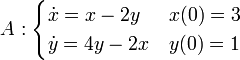$A:\begin{cases} \dot{x}=x-2y & x(0)=3\\ \dot{y}=4y-2x & y(0)=1\\ \end{cases}$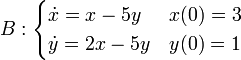$B:\begin{cases} \dot{x}=x-5y & x(0)=3\\ \dot{y}=2x-5y & y(0)=1 \end{cases}$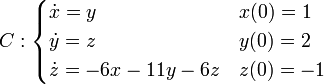$C:\begin{cases} \dot{x}=y & x(0)=1 \\ \dot{y}=z & y(0)=2 \\ \dot{z}=-6x-11y-6z & z(0)=-1 \end{cases}$

1. Write each one in a matrix form.
2. Find the eigenvalues and eigenvectors of the resulting matrices.
3. Diagonalize these matrices.
4. Compute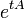$e^{tA}$ for each of those matrices.
5. Solve these equations.

1. Prove that if two matrices$A$ and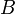$B$ satisfy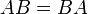$AB=BA$, then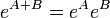$e^{A+B}=e^Ae^B$.
2. Find an example for two matrices$A$ and$B$ for which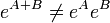$e^{A+B}\neq e^Ae^B$.
Task 3. Let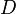$D$ be the differential operator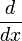$\frac{d}{dx}$, and let$f$ be a function of the variable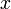$x$ whose Taylor series is convergent everywhere. Write a simple formula for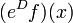$(e^Df)(x)$.
(Here, of course,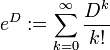$e^D:=\sum_{k=0}^\infty \frac{D^k}{k!}$).